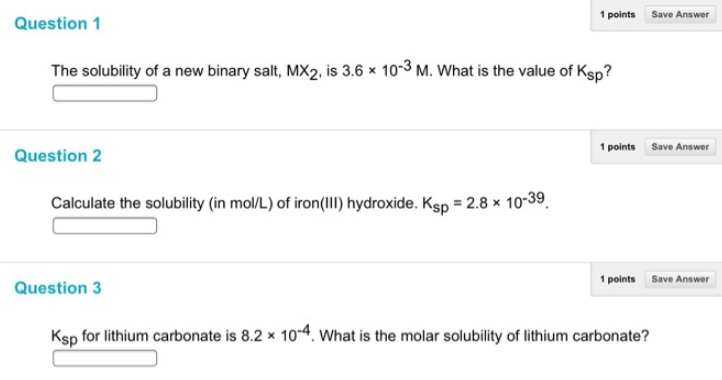# Problem: Question 1The solubility of a new binary salt, MX2, is 3.6 x 10-3 M. What is the value of Ksp? Question 2Calculate the solubility (in mol/L) of iron(Ill) hydroxide. Ksp = 2.8 x 10-39. Question 3Ksp for lithium carbonate is 8.2 x 10-4. What is the molar solubility of lithium carbonate?

###### FREE Expert Solution
86% (400 ratings)###### Problem Details

Question 1

The solubility of a new binary salt, MX2, is 3.6 x 10-3 M. What is the value of Ksp

Question 2

Calculate the solubility (in mol/L) of iron(Ill) hydroxide. Ksp = 2.8 x 10-39

Question 3

Ksp for lithium carbonate is 8.2 x 10-4. What is the molar solubility of lithium carbonate?Frequently Asked Questions

What scientific concept do you need to know in order to solve this problem?

Our tutors have indicated that to solve this problem you will need to apply the Ksp concept. You can view video lessons to learn Ksp. Or if you need more Ksp practice, you can also practice Ksp practice problems.